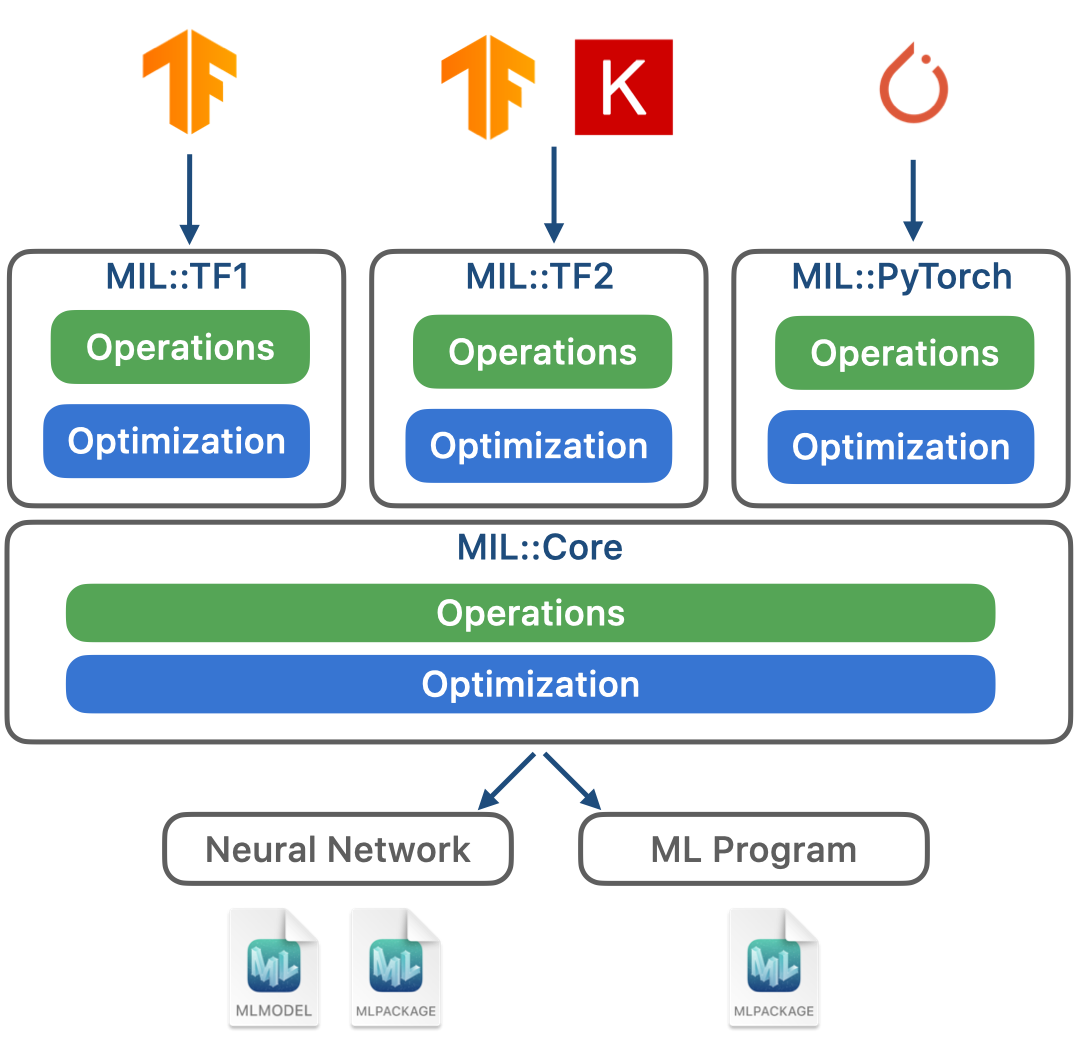## coremltools

Use Core ML to integrate machine learning models into your app. Core ML provides a unified representation for all models. Your app uses Core ML APIs and user data to make predictions, and to train or fine-tune models, all on the user’s device.

Core ML optimizes on-device performance by leveraging the CPU, GPU, and Neural Engine while minimizing its memory footprint and power consumption. Running a model strictly on the user’s device removes any need for a network connection, which helps keep the user’s data private and your app responsive.

# Model Intermediate Language

When converting a model to Core ML, coremltools first creates an intermediate representation of the model in the Model Intermediate Language (MIL), and then translates the MIL representation to the Core ML protobuf representation.

What can you do with MIL, and why would you need to use it? In most cases you define your networks in TensorFlow or PyTorch, and convert to Core ML using the Unified Conversion API. You don't need to know MIL except in the following use cases:

• If you get an unsupported operator error when converting the model, write a composite operator that uses the MIL builder described in this topic. See Composite Operators for details on writing a composite op.
• If you are defining a model from scratch rather than starting with TensorFlow or PyTorch, you can use MIL to define the model as described in "Create a MIL program" in this topic. You can use this technique to test a single op model.

# Overview

Behind the scenes, the converter takes a computation graph from a source framework such as TensorFlow or PyTorch, and builds the MIL program. The converter performs a series of optimizations with the MIL program and uses it to generate the MLModel.Rather than a single set of operations, MIL has multiple sets of operations and optimizations in order to accommodate source frameworks such as TensorFlow and PyTorch. For example, to convert a TensorFlow 1 model, you would use the operations and optimization tailored for TensorFlow 1 in the MIL::TF1 dialect, and coremltools converts them to the operations and optimization for the MIL::Core dialect.

# Create a MIL program

You can easily construct a MIL program using the Python Builder class for MIL as shown in the following example, which creates a program that takes as input (1, 100, 100, 3) and produces a few layers of the neural network. This program contains all the information needed to describe the neural network:

# import builder
from coremltools.converters.mil import Builder as mb

# Input to MIL program is a list of tensors. Here we have one input with
# shape (1, 100, 100, 3) and implicit dtype == fp32
@mb.program(input_specs=[mb.TensorSpec(shape=(1, 100, 100, 3)),])
def prog(x):
# MIL operation takes named inputs (instead of positional inputs).
# Here name argument is optional.
x = mb.relu(x=x, name='relu')
x = mb.transpose(x=x, perm=[0, 3, 1, 2], name='transpose')
x = mb.reduce_mean(x=x, axes=[2, 3], keep_dims=False, name='reduce')
x = mb.log(x=x, name='log')
return x

print(prog)


The output of the program is as follows:

main(%x: (1, 100, 100, 3, fp32)(Tensor)) {
block0() {
%relu: (1, 100, 100, 3, fp32)(Tensor) = relu(x=%x, name="relu")
%transpose_perm_0: (4,i32)*(Tensor) = const(val=[0, 3, 1, 2], name="transpose_perm_0")
%transpose: (1, 3, 100, 100, fp32)(Tensor) = transpose(x=%relu, perm=%transpose_perm_0, name="transpose")
%reduce_axes_0: (2,i32)*(Tensor) = const(val=[2, 3], name="reduce_axes_0")
%reduce_keep_dims_0: (bool)*(Scalar) = const(val=False, name="reduce_keep_dims_0")
%reduce: (1, 3, fp32)(Tensor) = reduce_mean(x=%transpose, axes=%reduce_axes_0, keep_dims=%reduce_keep_dims_0, name="reduce")
%log_epsilon_0: (fp32)*(Scalar) = const(val=1e-45, name="log_epsilon_0")
%log: (1, 3, fp32)(Tensor) = log(x=%reduce, epsilon=%log_epsilon_0, name="log")
} -> (%log)
}


In the above output, main is a MIL function. A MIL program contains one or more functions. The mb.program decorator creates a MIL program with a single function (main). The input to main is an fp32 tensor with the shape specified in the Python code.

Each function contains exactly one top-level block. The main function in the above example contains block0. The return values of the top-level block (%log) is the return value of the function.

A block is a sequence of operations. The above example shows 10 operations in block0. Five of them are const operations that produces constants such as the permutation order (%transpose_perm_0).

# Convert MIL to Core ML

You can translate the MIL representation to the Core ML protobuf representation for either a neural network or an ML program. (For a comparison, see Comparing ML programs and neural networks.)

To convert to an ML program, use the following code:

model = ct.convert(prog, convert_to="mlprogram")


To convert to a neural network, use the following:

model = ct.convert(prog)


For example, you can convert the MIL program from the previous section to a neural network, and then run a prediction with the converted model:

# Note: This example continues the code from the previous section.
import coremltools as ct
import numpy as np

# Convert to CoreML neural network format
model = ct.convert(prog)

# Make a prediction with CoreML
prediction = model.predict({
'x': np.random.rand(1, 100, 100, 3).astype(np.float32),
})


The example in this topic uses MIL operations such as mb.relu and mb.transpose. To learn which operations MIL supports and their parameters, see the API Reference for converters.mil.ops. You can find the details for each operation in the source code for MIL ops.Updated about a year ago